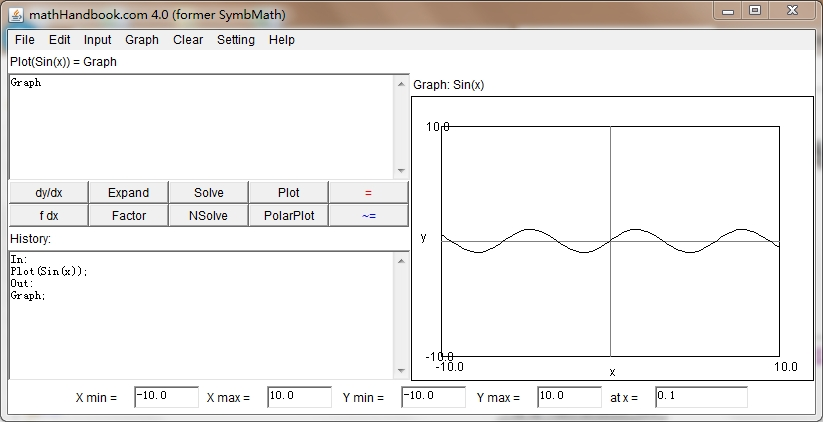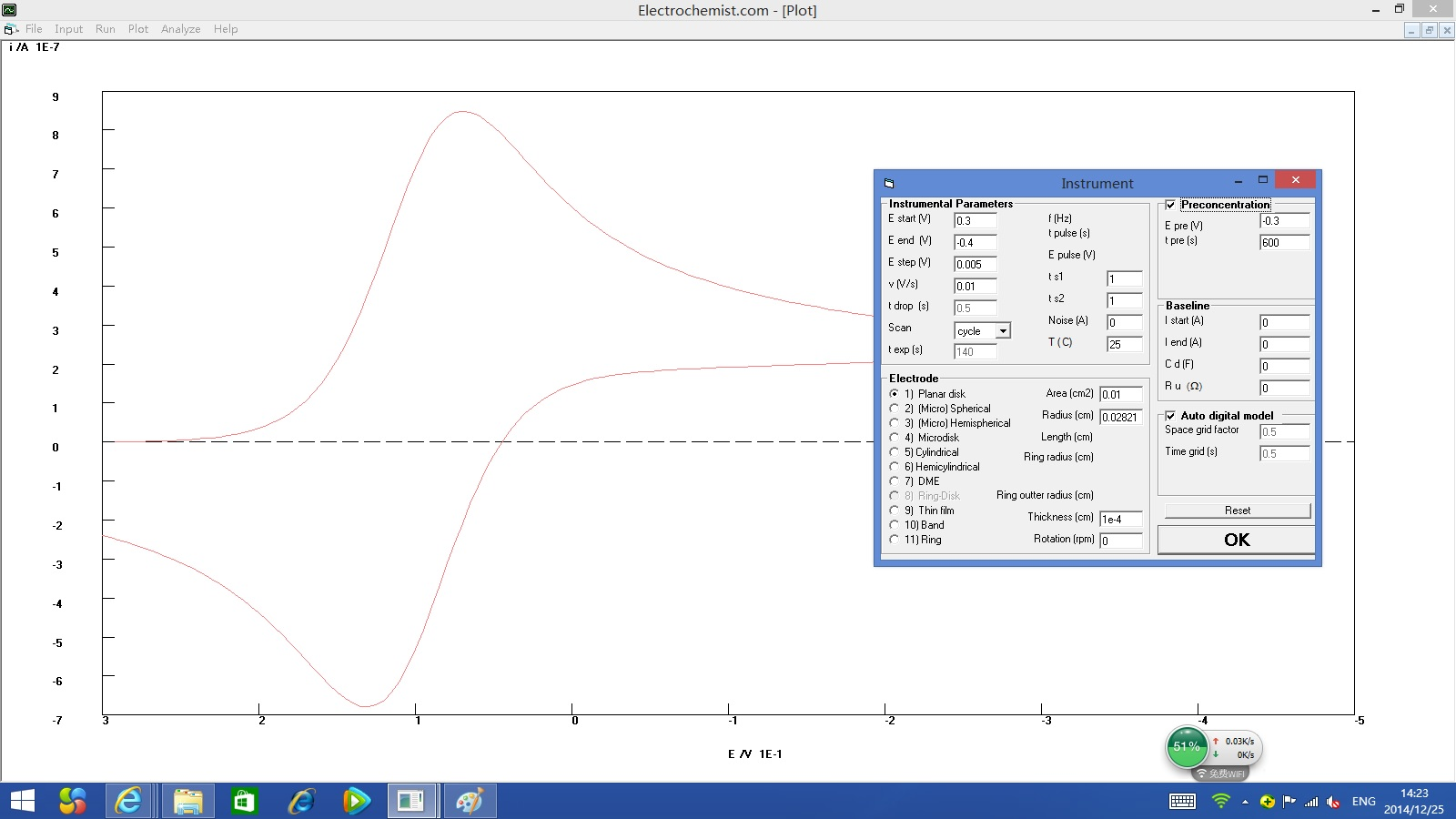﻿ ﻿﻿ Fractional Calculus Computer Algebra System math software Home | list | math | function | calculator | handbook | help | forum | contact | index | 中文﻿

Gamma gamma

Gamma and incomplete Gamma functions

smallgamma

small gamma and incomplete small gamma functions

loggamma

log gamma function

lngamma

log gamma function

Calling Sequence Parameters Description Examples References Source

Calling Sequence

Gamma(a) Γ(a)
Gamma(a, z) Γ(a,z)
gamma(n,b, z) gamma(n,b,z)
smallgamma(a, z) gamma(a,0,z)
loggamma(a) logΓ(a)

a - power
b - lower bond
z - upper bond

Description

• The Gamma function is defined for Re(z)>0 by
Γ(z)= int_0^oo e^(-t) t^(z-1) dt
and is extended to the rest of the complex plane, less the non-positive integers, by analytic continuation. Gamma has a simple pole at each of the points z=0,-1,-2,....

For Re(a)>0, we also have the integral representation
Γ(a,z)=int_z^oo e^(-t) t^(a-1) dt
gamma(a,0,z)=int_0^z e^(-t) t^(a-1) dt = Γ(a)−Γ(a,z)
gamma(a,c,z)=int_c^z e^(-t) t^(a-1) dt = Γ(a,c)−Γ(a,z)
Some authors refer to it's incomplete Gamma function as the complementary or upper incomplete gamma function, and call smallgamma(a,x)=Gamma(a)-Gamma(a,z) as the incomplete or lower incomplete small gamma function.
• The incomplete Gamma function is defined as:
Γ(a,z)=Γ(a)−za 1F1(a,1+a,−z)a
where 1F1 is the confluent hypergeometric function (in it notation, 1F1(a,1+a,-z) = hypergeom([a],[1+a],-z)).
• The gamma function extends the classical factorial function to the complex plane: gamma( n ) = (n-1)!. In general, it does not distinguish these two functions, although the factorial function will evaluate for any positive integer, while for integer n, gamma(n) will evaluate only if n is not too large. Use expand to force gamma(n) to evaluate.
• You can enter the command gamma using either the 1-D or 2-D calling sequence. For example, gamma(5) is equivalent to Γ(5).
• For positive real arguments z, the lngamma function is defined by:
lnΓ(z)=ln(Γ(z))
For complex z, it evaluates the principal branch of the log-gamma function, which is defined by analytic continuation from the positive real axis. Each of the points z=0,-1,-2,..., is a singularity and a branch point, and the union of the branch cuts is the negative real axis. On the branch cuts, the values of lngamma(z) are determined by continuity from above. (Note, therefore, that lngamma <> ln(gamma(z)) in general.)

Examples


>   Γ(1)
1
(1)

>   Γ(5)=4!
24=24
(2)

>   Γ(−1.4)=(−2.4)!
2.659271873=2.659271873
(3)

>   Γ(4,−1)
2e
(4)

>   Γ(1.0+2.5I)
0.06687277236+0.04032263512I
(5)

>   Γ(1.0+2.5I,2.0+3.5I)
0.01314614269+0.006253182683I
(6)

>   loggamma(1.234+2.345I)
−2.132556911+0.7097892285I
(7)

>   lngamma(−1.5)≠ln(Γ(−1.5))
0.8600470154−6.283185307I≠0.8600470153

(8)

References

Erdelyi, A. Higher Transcendental Functions. McGraw-Hill, 1953.
Hare, D. E. G. "Computing the Principal Branch of log-gamma." Journal of Algorithms, (November 1997): 221-236.


﻿